## Science

• builds and organizes knowledge
• tests explanations about the universe
• systematically,
• objectively,
• transparently,
• and reproducibly.

Otherwise it's not science.

## Computers

### should...

• improve efficiency,
• reduce human error,
• automate the mundane,
• simplify the complex,
• and accelerate research.

But scientists don't use them effectively.

“ Computational science is a special case of scientific research: the work is easily shared via the Internet since the paper, code, and data are digital and those three aspects are all that is required to reproduce the results, given sufficient computation tools. ” - Stodden, 2010.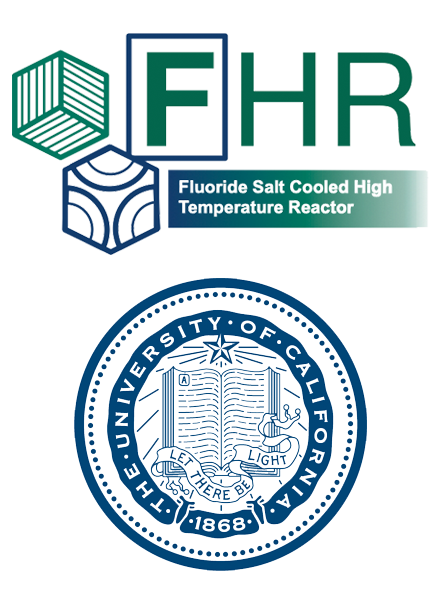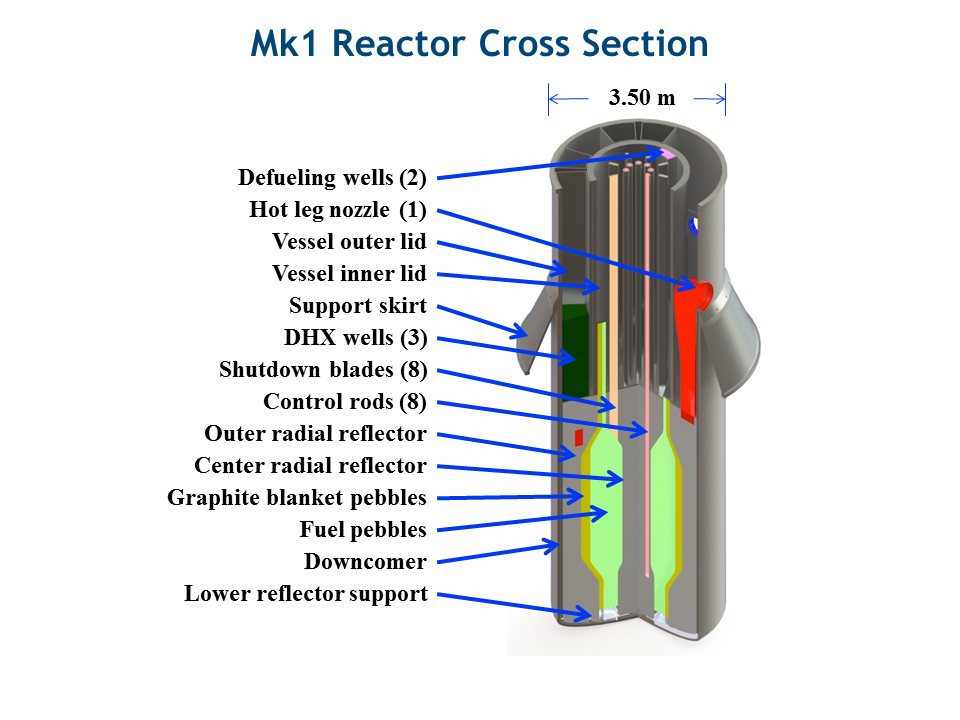## PB-FHR Fuel Geometry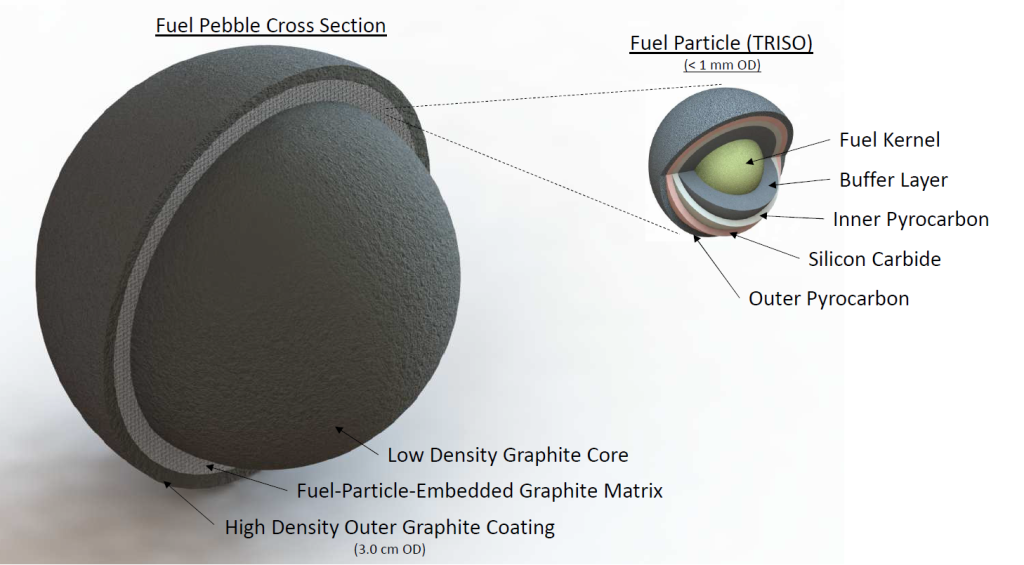## Coupled Multi-Physics Analysis

Severe accident neutronics and thermal hydraulics can be simulated beautifully for simple geometries and well studied materials. (below, INL BISON work.)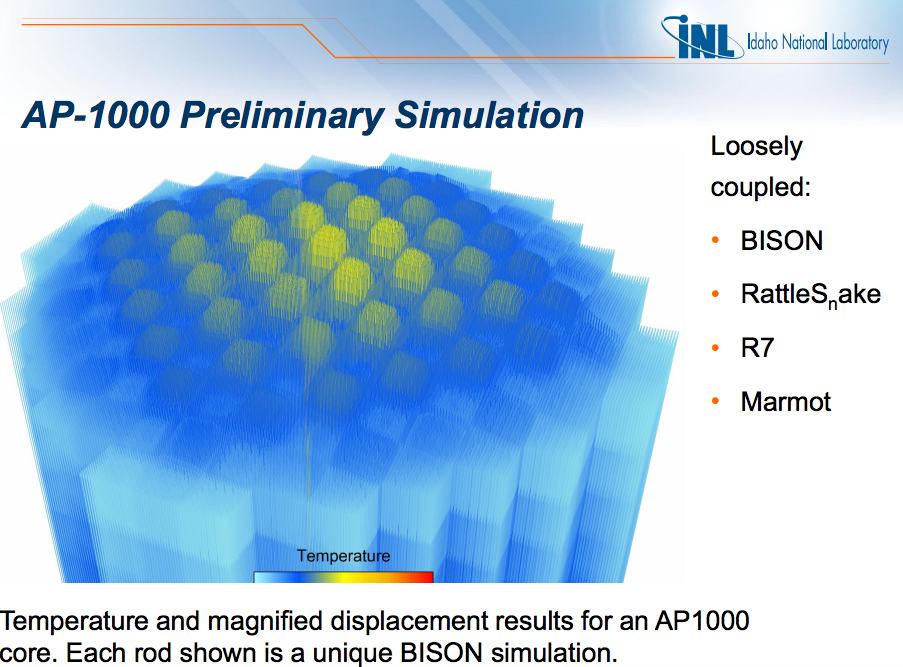## FHR, Accident Transient Analysis

• Collect experimental data
• Conduct algebraic, static, and benchmark simulations
• Develop 0D coupled neutronics/TH model (PyRK)
• Develop 3D neutronics/TH model
• Compare 0D and 3D simulations
• Couple additional physics (e.g. fuel performance)PyRK: Python for Reactor Kinetics

## Review of Nuclear Reactor Kinetics$\sigma(E,\vec{r},\hat{\Omega},T,x,i)$$k=1$

## Reactivity

\begin{align} k &= \mbox{"neutron multiplication factor"}\\ &= \frac{\mbox{neutrons causing fission}}{\mbox{neutrons produced by fission}}\\ \rho &= \frac{k-1}{k}\\ \rho &= \mbox{reactivity}\\ \end{align}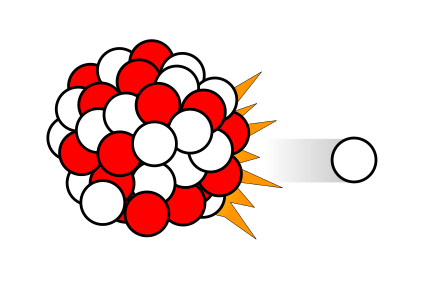$\beta_i, \lambda_{d,i}$

## PyRK

• 6-precursor-group,
• 11-decay-group Point Reactor Kinetics model
• Lumped Parameter thermal hydraulics model
• Object-oriented, geometry and material agnostic framework

## Point Reactor Kinetics

\begin{align} p &= \mbox{ reactor power }\\ \rho(t,&T_{fuel},T_{cool},T_{mod}, T_{refl}) = \mbox{ reactivity}\\ \beta &= \mbox{ fraction of neutrons that are delayed}\\ \beta_j &= \mbox{ fraction of delayed neutrons from precursor group j}\\ \zeta_j &= \mbox{ concentration of precursors of group j}\\ \lambda_{d,j} &= \mbox{ decay constant of precursor group j}\\ \Lambda &= \mbox{ mean generation time }\\ \omega_k &= \mbox{ decay heat from FP group k}\\ \kappa_k &= \mbox{ heat per fission for decay FP group k}\\ \lambda_{FP,k} &= \mbox{ decay constant for decay FP group k}\\ T_i &= \mbox{ temperature of component i} \end{align}
$\frac{d}{dt}\left[ \begin{array}{c} p\\ \zeta_1\\ .\\ \zeta_j\\ .\\ \zeta_J\\ \omega_1\\ .\\ \omega_k\\ .\\ \omega_K\\ T_{i}\\ .\\ T_{I}\\ \end{array} \right] = \left[ \begin{array}{ c } \frac{\rho(t,T_{i},\cdots)-\beta}{\Lambda}p + \displaystyle\sum^{j=J}_{j=1}\lambda_{d,j}\zeta_j\\ \frac{\beta_1}{\Lambda} p - \lambda_{d,1}\zeta_1\\ .\\ \frac{\beta_j}{\Lambda}p-\lambda_{d,j}\zeta_j\\ .\\ \frac{\beta_J}{\Lambda}p-\lambda_{d,J}\zeta_J\\ \kappa_1p - \lambda_{FP,1}\omega_1\\ .\\ \kappa_kp - \lambda_{FP,k}\omega_k\\ .\\ \kappa_{k p} - \lambda_{FP,k}\omega_{k}\\ f_{i}(p, C_{p,i}, T_{i}, \cdots)\\ .\\ f_{I}(p, C_{p,I}, T_{I}, \cdots)\\ \end{array} \right]$

## Lumped Parameter Heat Transfer

The heat flow out of body $i$ is the sum of surface heat flow by conduction, convection, radiation, and other mechanisms to each adjacent body, $j$: \begin{align} Q &= Q_i + \sum_j Q_{ij}\\ &=Q_i + \sum_j\frac{T_{i} - T_{j}}{R_{th,ij}}\\ \dot{Q} &= \mbox{total heat flow out of body i }[J\cdot s^{-1}]\\ Q_i &= \mbox{other heat transfer, a constant }[J\cdot s^{-1}]\\ T_i &= \mbox{temperature of body i }[K]\\ T_j &= \mbox{temperature of body j }[K]\\ j &= \mbox{adjacent bodies }[-]\\ R_{th} &= \mbox{thermal resistence of the component }[K \cdot s \cdot J^{-1}]. \end{align}

## Quality Control

“ Organized Skepticism. Scientists are critical: All ideas must be tested and are subject to rigorous structured community scrutiny.” - R.K. Merton, 1942

## Unit Checking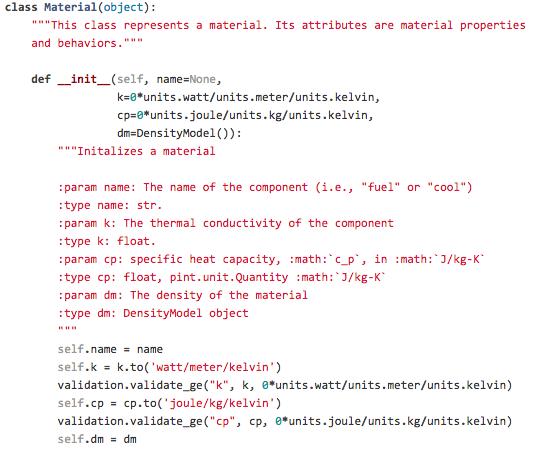In PyRK, the Pint package (pint.readthedocs.org/en/0.6/) is used keeping track of units, converting between them, and throwing errors when unit conversions are not sane.

## Backing Up Files

• Good: hope
• Better: nightly emails
• Best: remote version control

Version Control Systems: cvs, svn, hg, git

## Managing Changes

• Good: naming convention
• Better: clever naming convention
• Best: local version control

## Version Control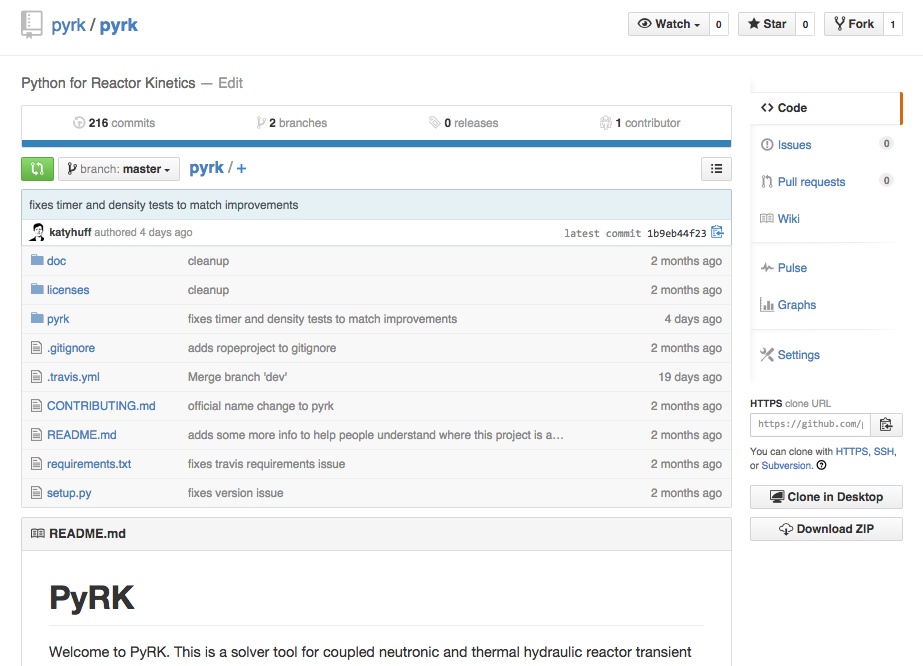Keeping track of versions of the code makes it possible to experiment without fear and placing the code online encourages use and collaboration.

## Automated Documentation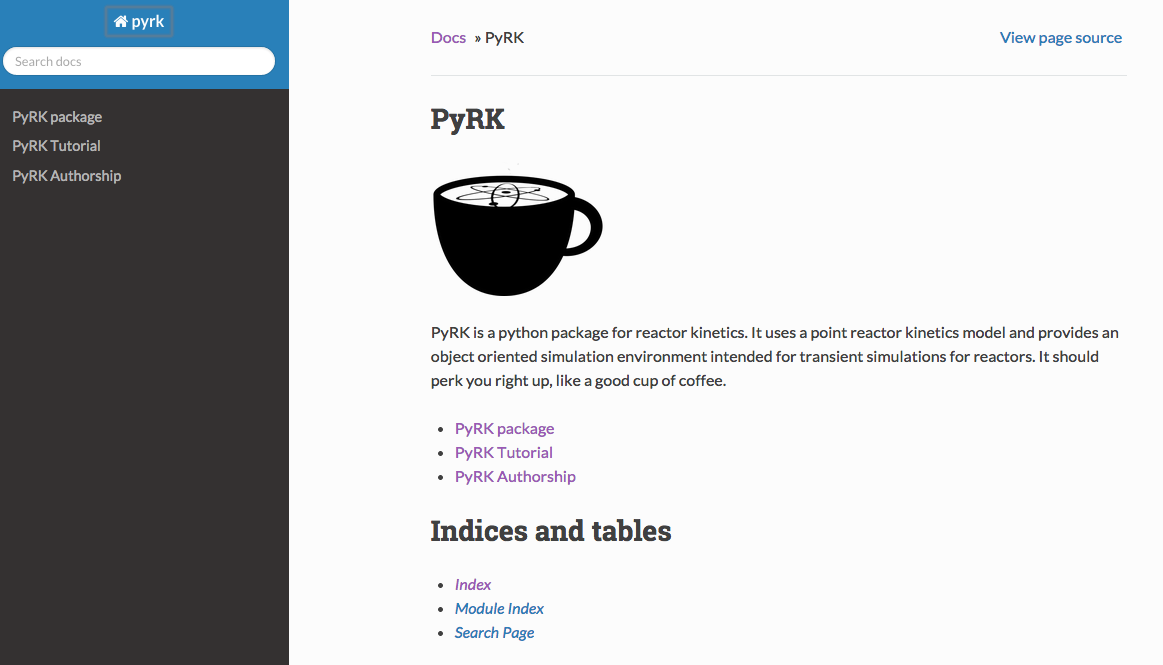Automated documentation creates a browsable website explaining the most recent version of the code.

## Error Detection

“ The scientific method’s central motivation is the ubiquity of error—the awareness that mistakes and self-delusion can creep in absolutely anywhere and that the scientist’s effort is primarily expended in recognizing and rooting out error. ” - Donoho, 2009.

## Error Detection

• Good: show results to experts
• Better: integration testing
• Best: unit test suite, continuous integration

## Test Suite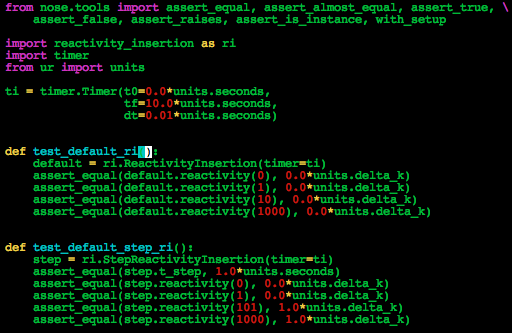The classes and functions that make up the code are tested individually for robustness using nose.

## Continuous Integration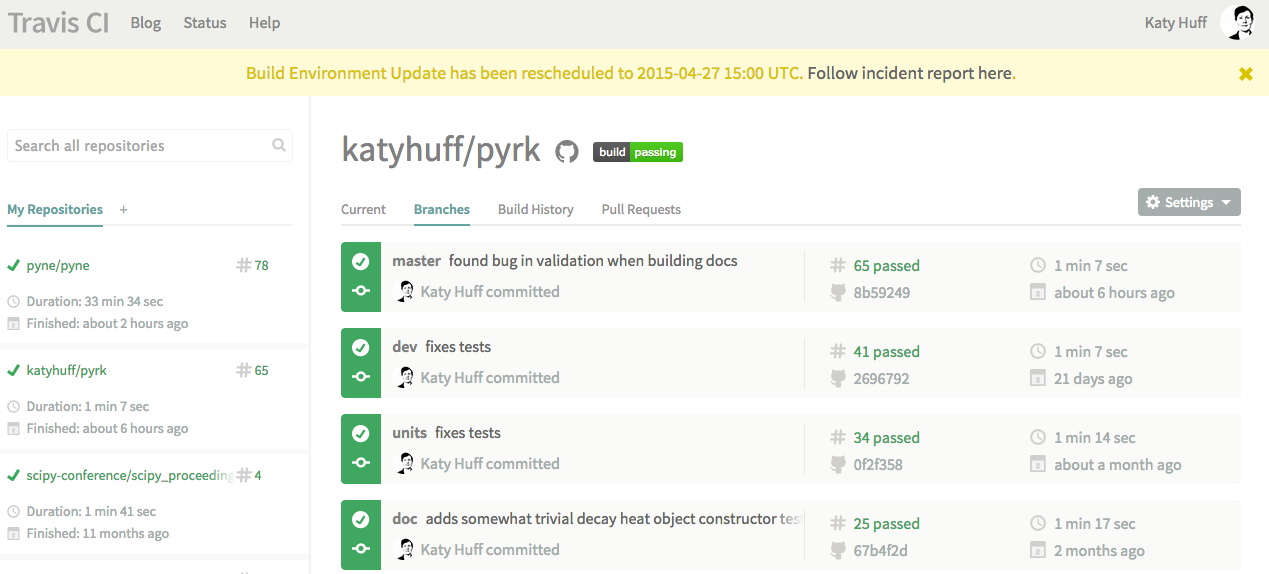The tests are run every time a change is made to the repository online. The results are public. If a main branch has a failed test, I get an email.

### PB-FHR Reactivity Insertion

Pebble-Bed, Fluoride Salt Cooled, High-Temperature Reactor
• Molten FLiBe Coolant
• Annular Core
• Annular Pebble Fuel
• Ramp Reactivity Insertion, 600pcm over 10s

### Ramp Reactivity Insertion


# External Reactivity
from reactivity_insertion import RampReactivityInsertion
rho_ext = RampReactivityInsertion(timer=ti,
t_start=t_feedback + 10.0*units.seconds,
t_end=t_feedback + 20.0*units.seconds,
rho_init=0.0*units.delta_k,
rho_rise=600.0*units.pcm,
rho_final=600.0*units.pcm)


### Components



fuel = th.THComponent(name="fuel",
mat=TRISO(),
vol=vol_fuel,
T0=t_fuel,
alpha_temp=alpha_fuel,
timer=ti,
heatgen=True,
power_tot=power_tot/n_pebbles,
sph=True,
ri=r_mod,
ro=r_fuel
)

mod = th.THComponent(name="mod",
mat=Graphite(),
vol=vol_mod,
T0=t_mod,
alpha_temp=alpha_mod,
timer=ti,
sph=True,
ri=0.0,
ro=r_mod)

cool = th.THComponent(name="cool",
mat=Flibe(),
vol=vol_cool,
T0=t_cool,
alpha_temp=alpha_cool,
timer=ti)

shell = th.THComponent(name="shell",
mat=Graphite(),
vol=vol_shell,
T0=t_shell,
alpha_temp=alpha_shell,
timer=ti,
sph=True,
ri=r_fuel,
ro=r_shell)


### Heat Transfer


# The coolant convects to the pebbles


### Ramp Reactivity Insertion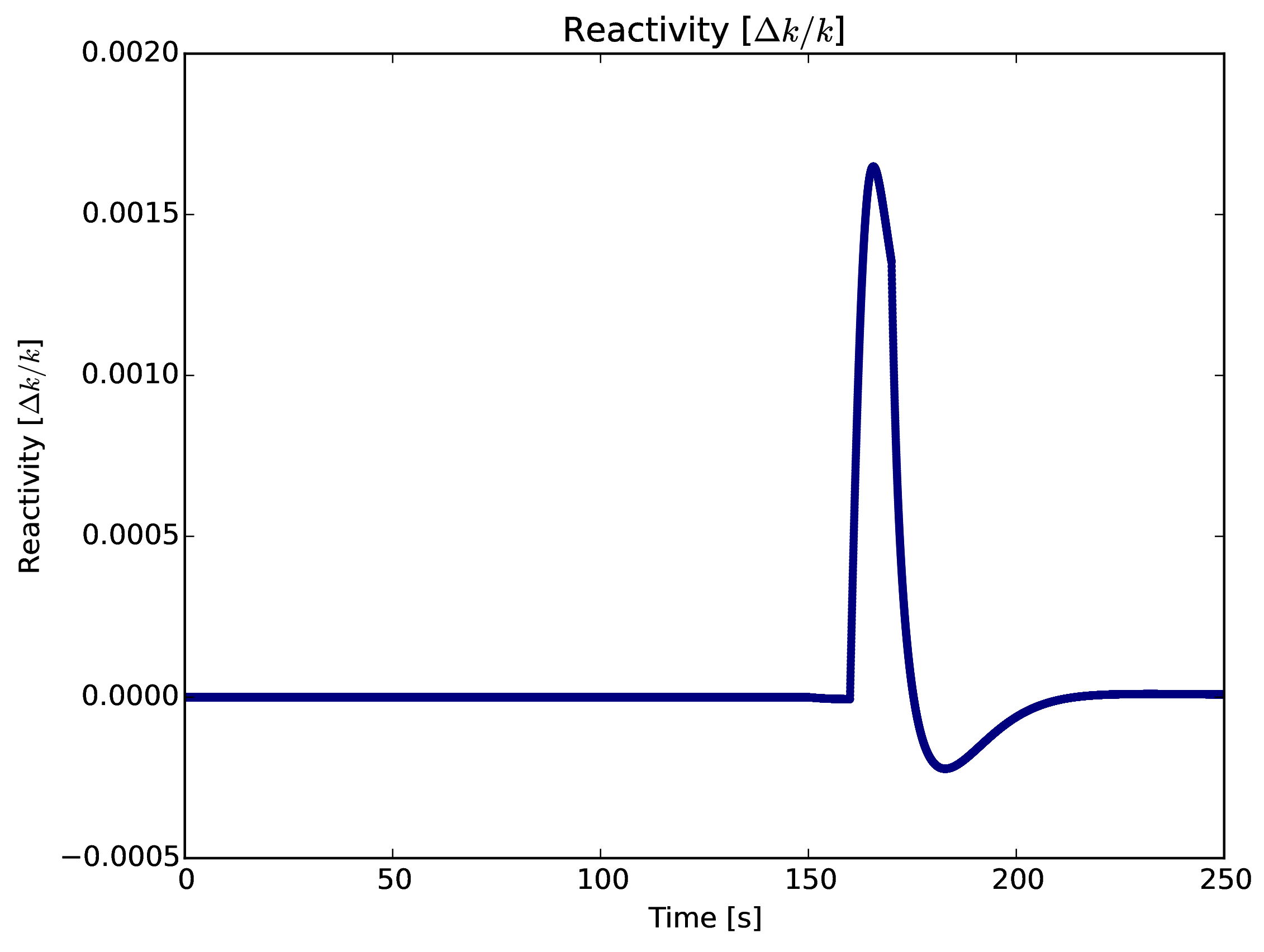### Fuel Layer Temperature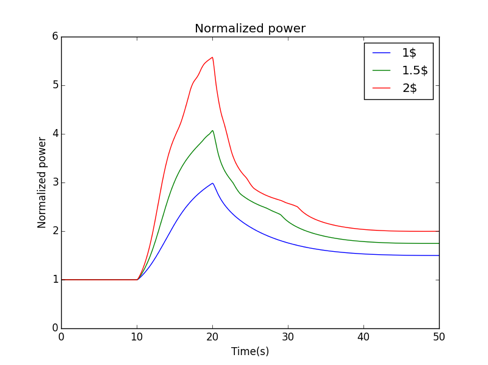### Coolant Temperature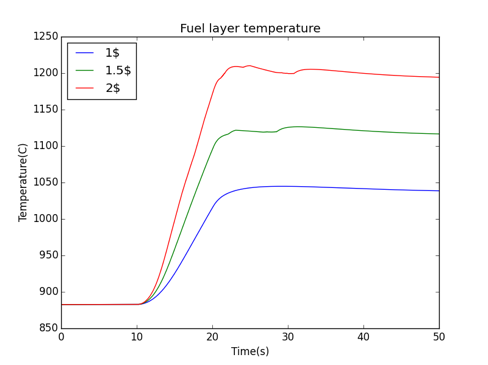## The Nuclear Fuel Cycle

Hundreds of discrete facilities mine, mill, convert, fabricate, transmute, recycle, and store nuclear material.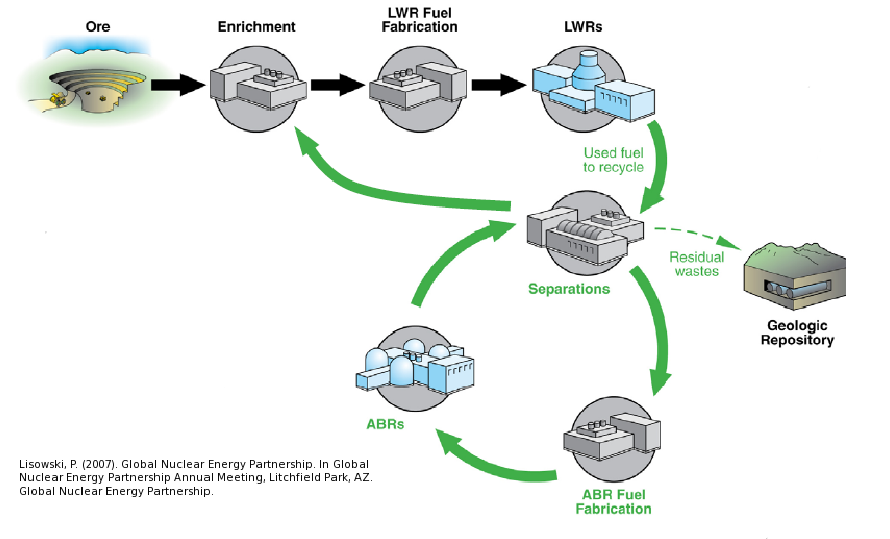## Fuel Cycle Metrics

• Mass Flow
• proliferation resistance and physical protection (PRPP) indices.
• Cost
• levelized cost of electricity,
• facility life cycle costs.
• Economics
• power production, facility deployments,
• dynamic pricing and feedback.
• Disruptions
• reliability, safety,
• system robustness.

### Current Simulators

• CAFCA (MIT)
• COSI (CEA)
• DANESS (ANL)
• DESAE (Rosatom)
• Evolcode (CIEMAT)
• FAMILY (IAEA)
• GENIUSv1 (INL)
• GENIUS v2 (UW)
• NFCSim (LANL)
• NFCSS (IAEA)
• NUWASTE (NWTRB)
• ORION (NNL)
• MARKAL (BNL)
• VISION (INL)

## State of the Art

### Performance

• Speed interactive time scales
• Fidelity: detail commensurate with existing challenges
• Detail: discrete material and agent tracking
• Regional Modeling: enabling international socio-economics

## Beyond the State of the Art

### Access

• Openness: for collaboration, validation, and code sustainability.
• Usability: for a wide range of user sophistication

### Extensibility

• Modularity: core infrastructure independent of proprietary or sensitive data and models
• Flexibility with a focus on robustness for myriad potential developer extensions.

## Extensibility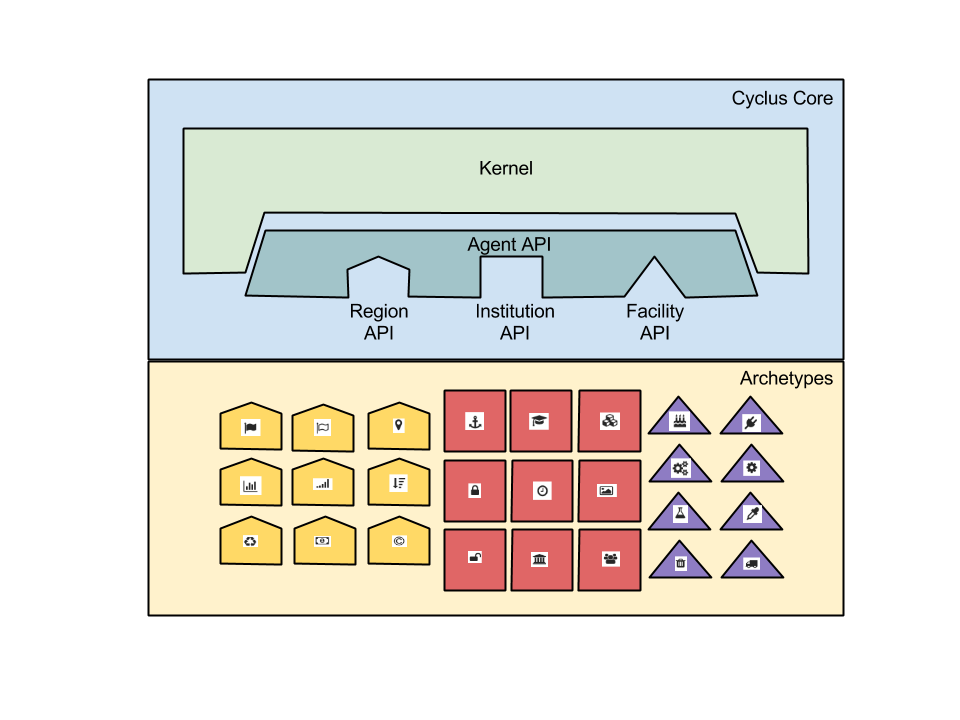## Openness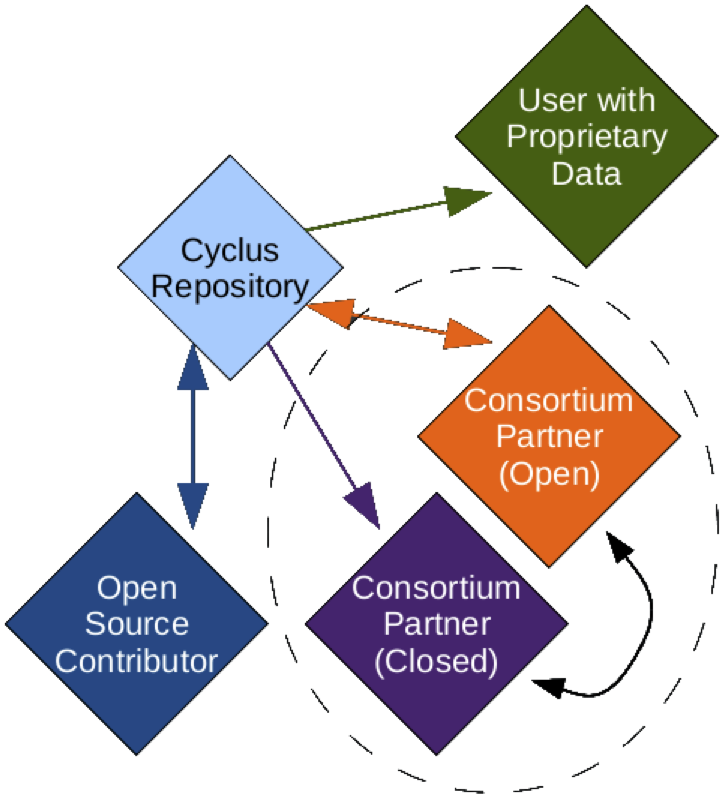## Growing Ecosystem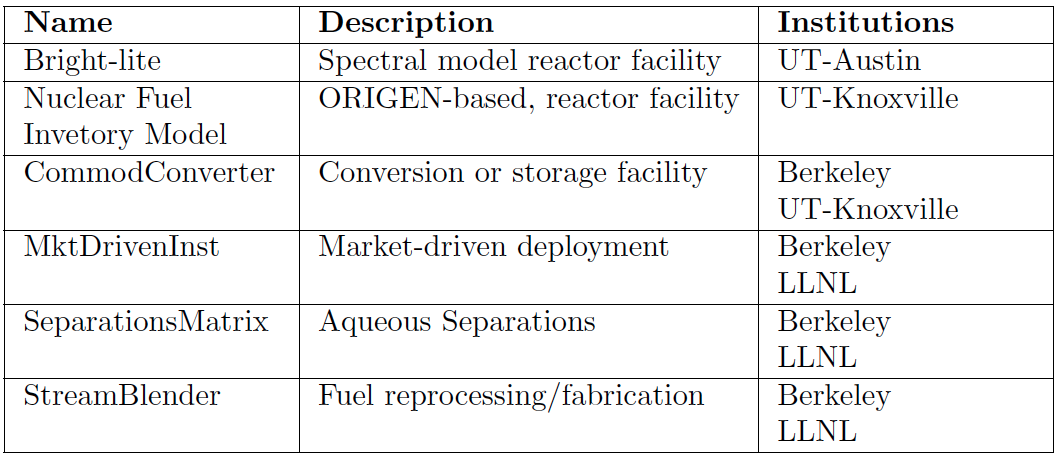## ...Well Beyond

### Algorithmic Sophistication

• Efficient: memory-efficient isotope tracking
• Customizable: constrained fuel supply
• Dynamic: isotopic-quality-based resource routing
• Physics-based: fuel fungibility

## Agent Based Systems Analysis

An agent-based simulation is made up of actors and communications between those actors.## Agent Based Systems Analysis

A facility might create material.## Agent Based Systems Analysis

It might request material.## Agent Based Systems Analysis

It might do both.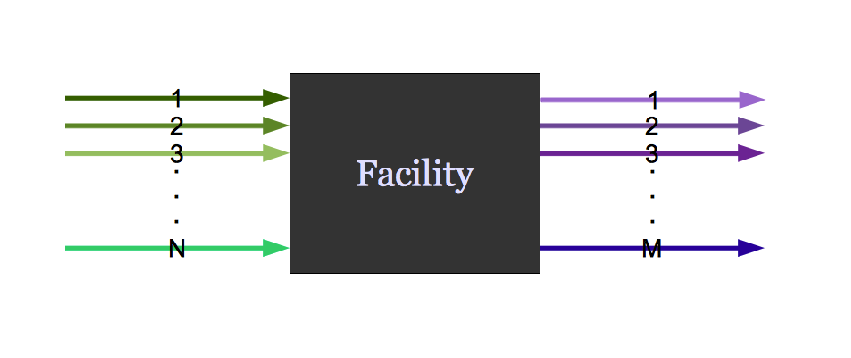## Agent Based Systems Analysis

Even simple fuel cycles have many independent agents.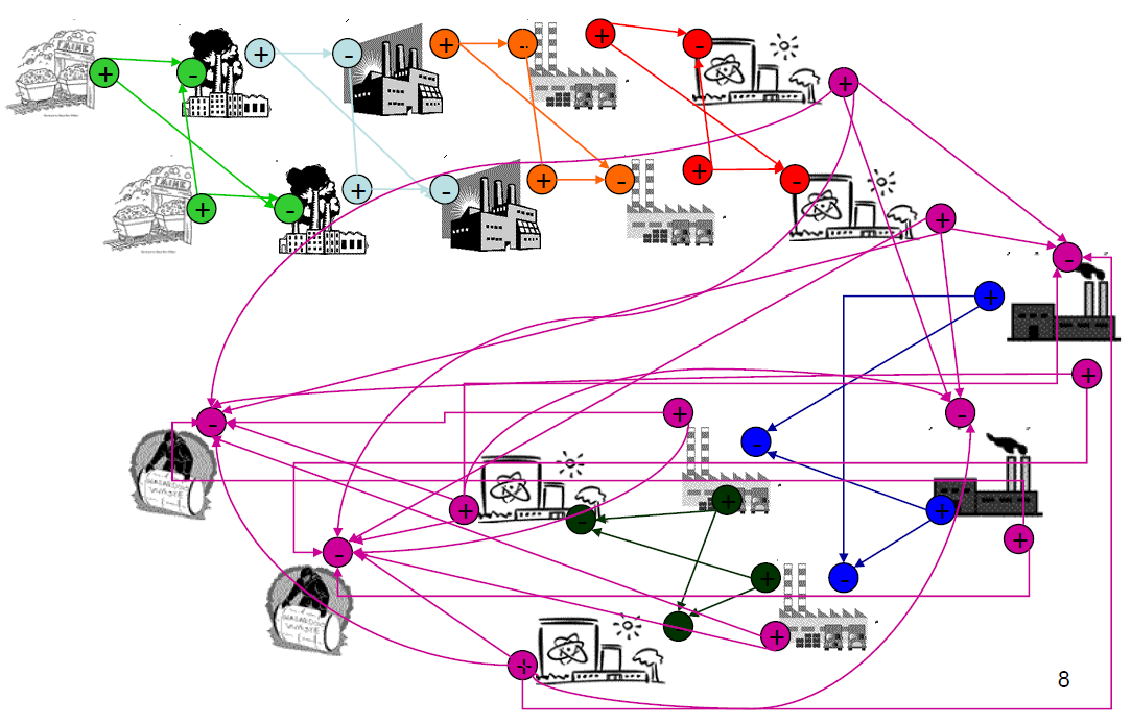## Dynamic Resource Exchange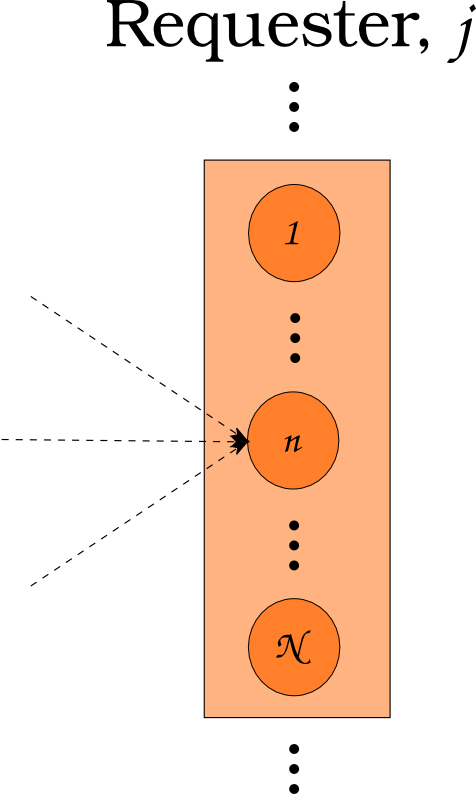$N_i \subset N$

## Dynamic Resource Exchange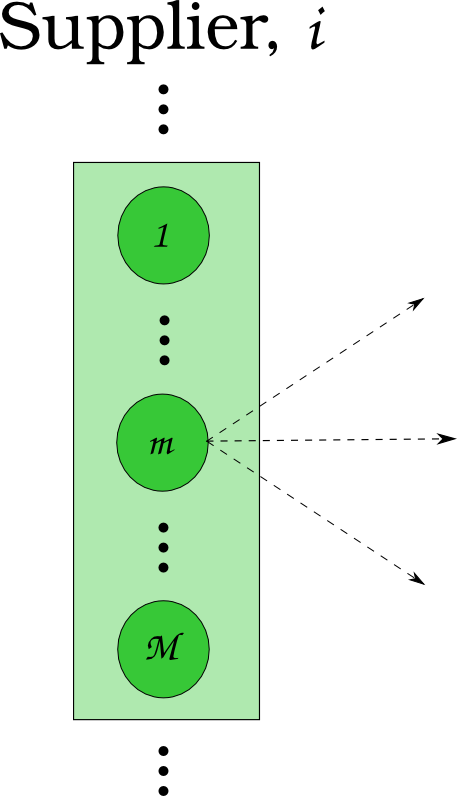$N_j \subset N$

## Dynamic Resource Exchange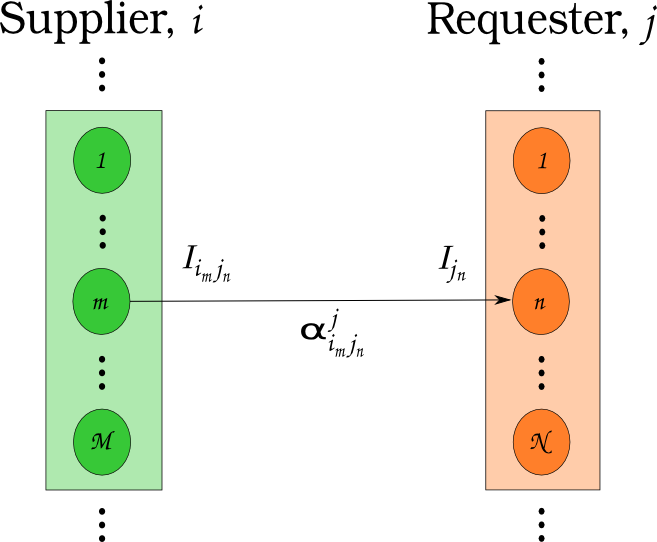$N_i \cup N_j = N$

## Feasibility vs. Optimization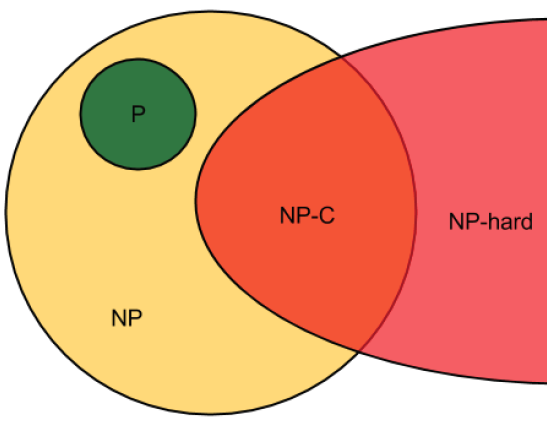If a decision problem is in NP-C, then the corresponding optimization problem is NP-hard.

## Multi-Commodity Transportation Formulation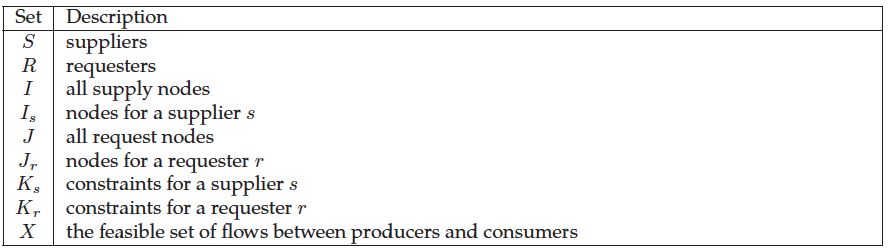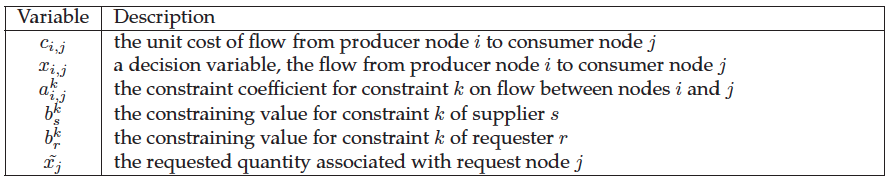## Multi-Commodity Transportation Formulation

\begin{align} \min_{x} z &= \sum_{i\in I}\sum_{j\in J} c_{i,j}x_{i,j} & \\ s.t & \sum_{i\in I_s}\sum_{j\in J} a_{i,j}^k x_{i,j} \le b_s^k & \forall k\in K_s, \forall s\in S\\ & \sum_{J\in J_r}\sum_{i\in I} a_{i,j}^k x_{i,j} \le b_r^k & \forall k\in K_r, \forall r\in R\\ & x_{i,j} \in [0,x_j] & \forall i\in I, \forall j\in J \end{align}

## Dynamic Resource Exchange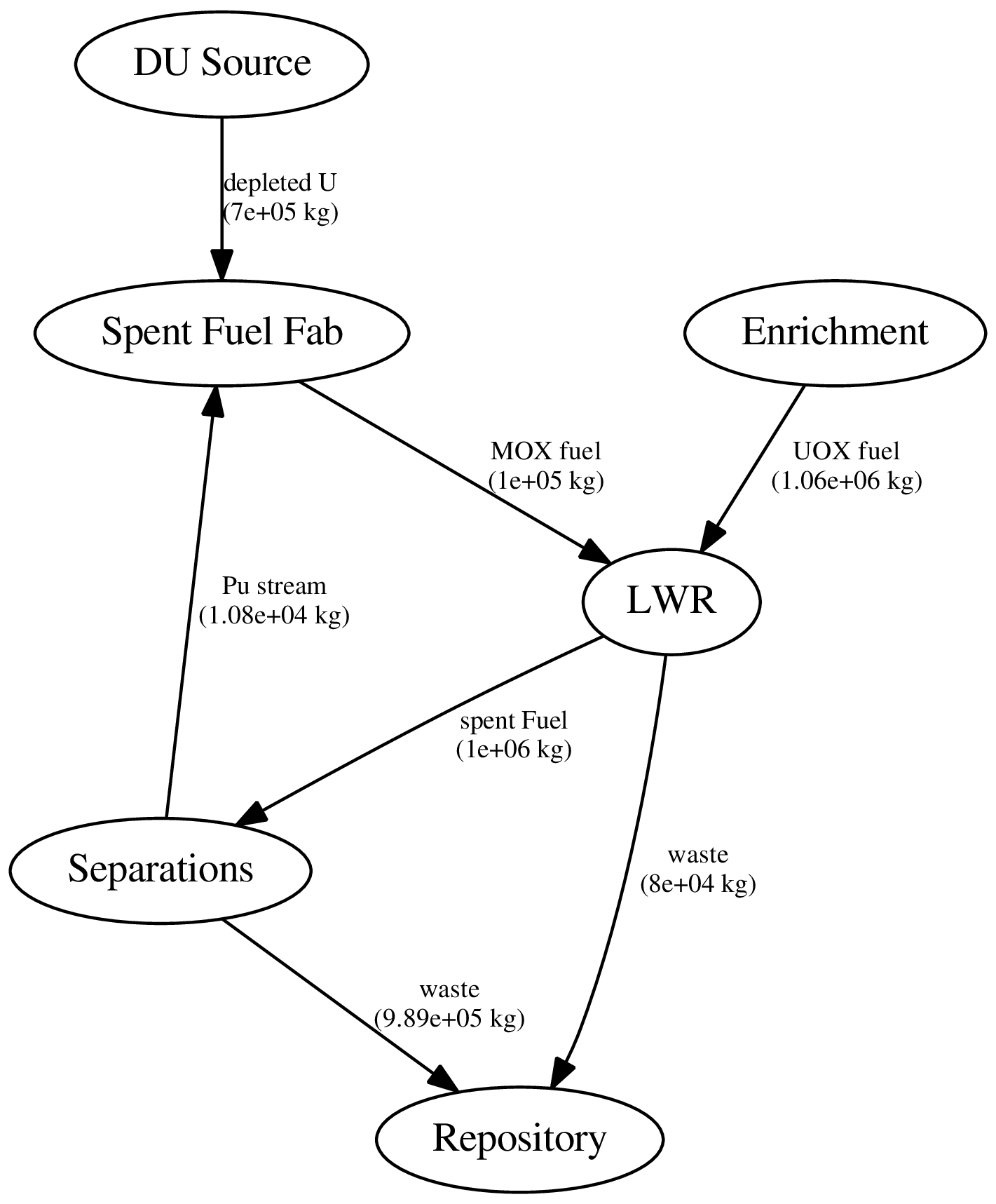## Dynamic Resource Exchange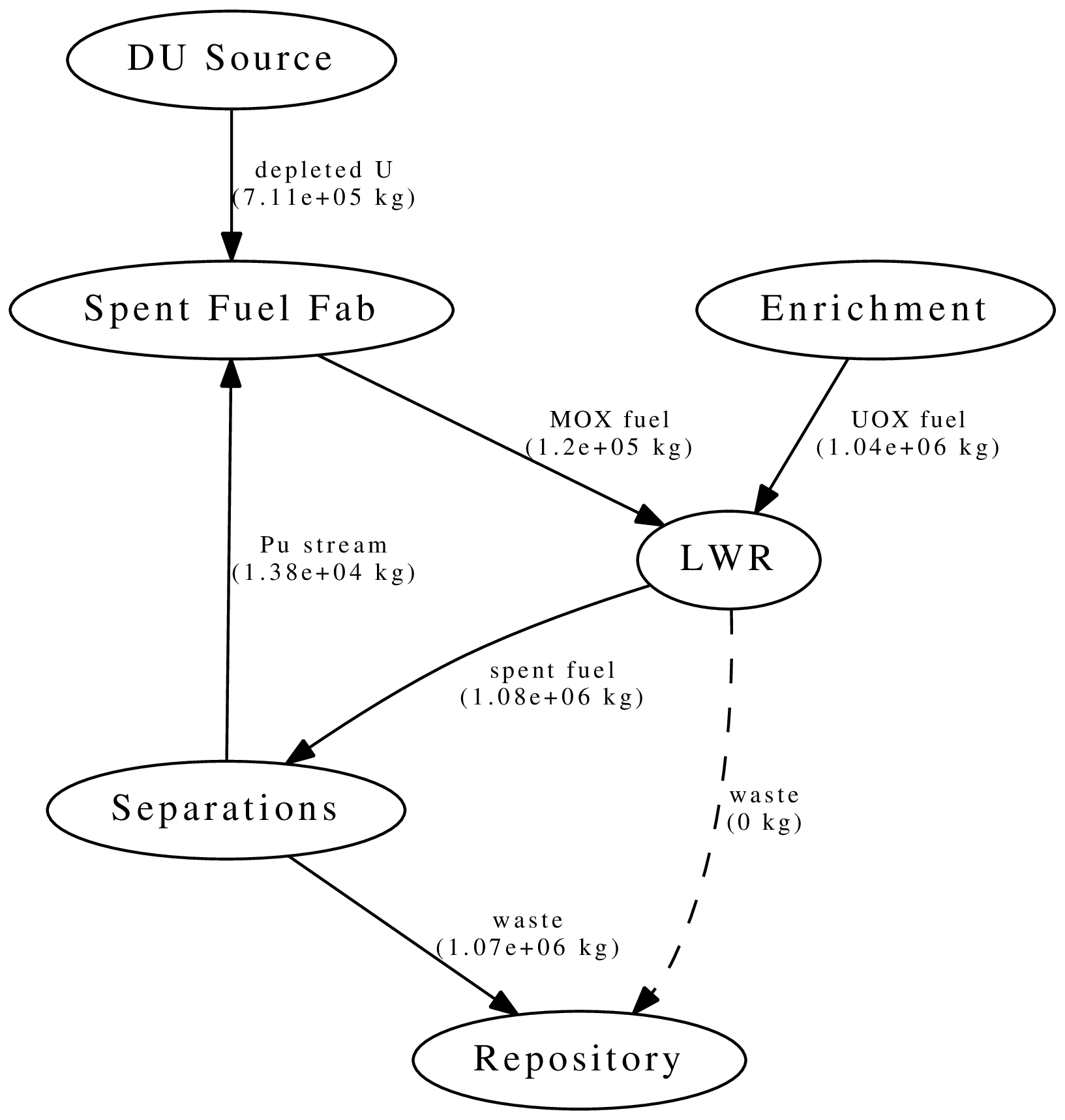## Dynamic Resource Exchange



mox
-    waste
+    spent_fuel
mox_fresh_fuel
mox_spent_fuel



## Dynamic Resource Exchange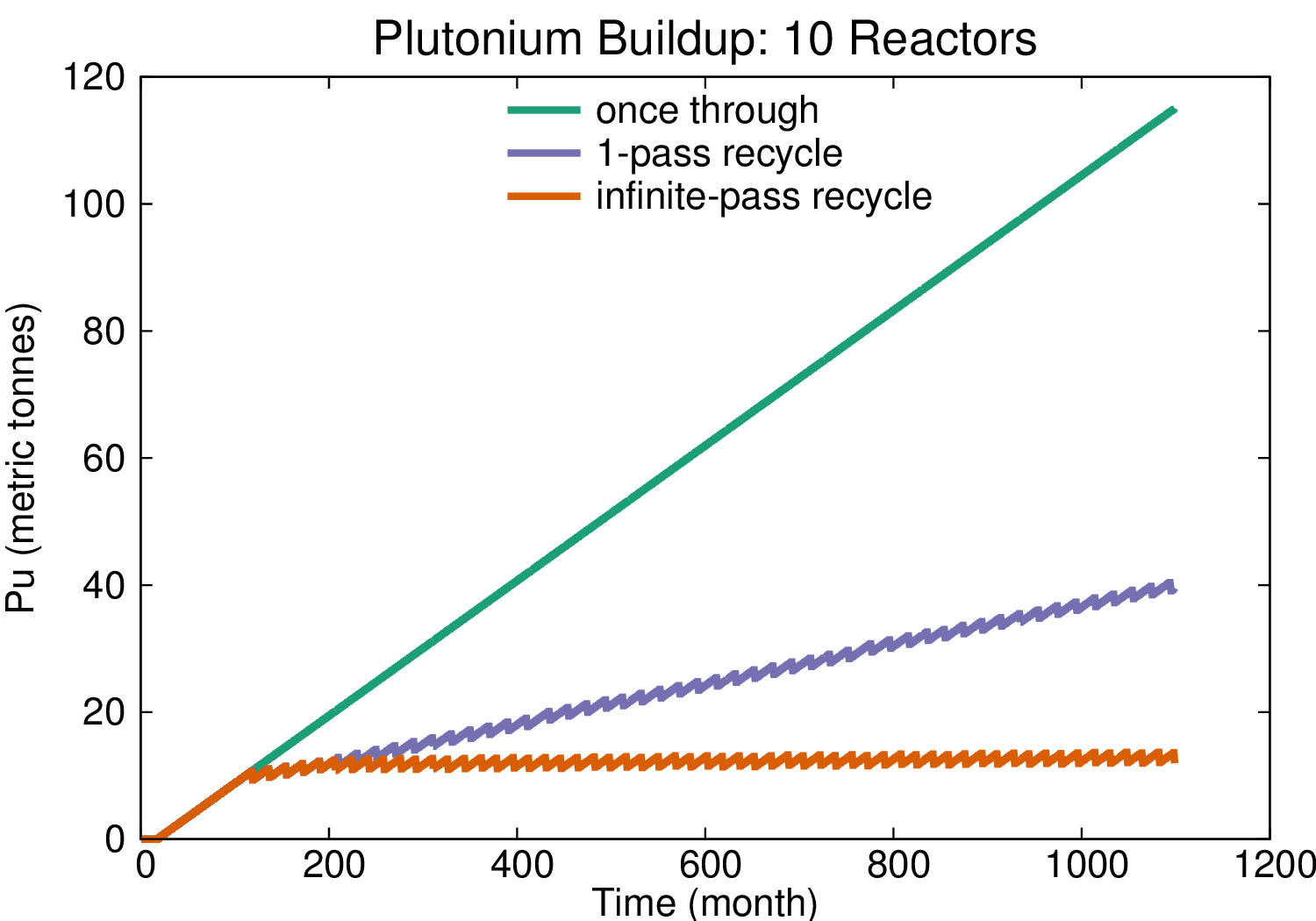## Transition Analysis

• LWR to SFR
• $T_0 = 2015$
• $T_f <= 2215$
• $C_0 = 100$ GWe LWR
• Annual nuclear energy demand growth: 1%
• Spent LWR fuel reprocessed to fabricate FR fuel
• Spent FR fuel reprocessed to fabricate FR fuel

## Transition Analysis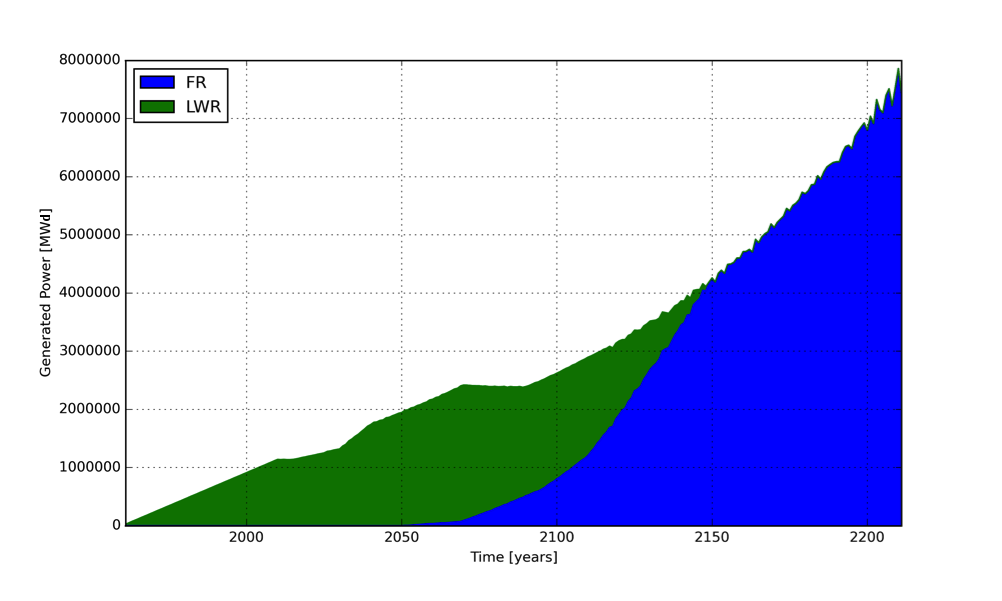Power generated by reactor type.

## Transition Analysis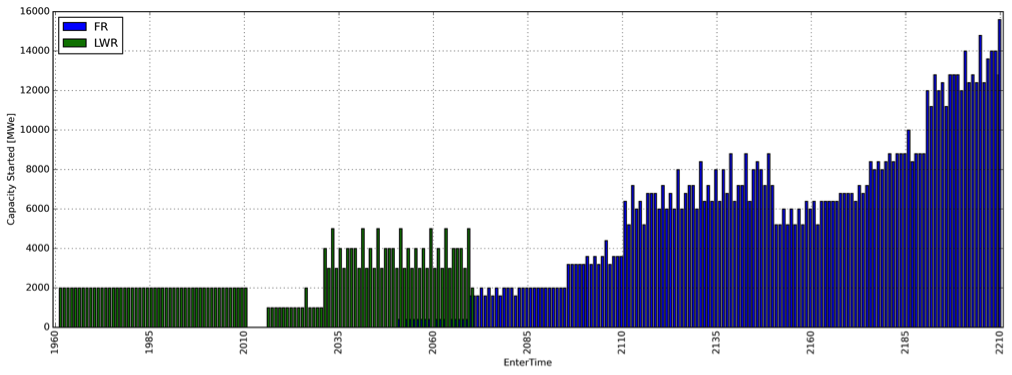Capacity deployed each year, by reactor type.

# Resources

Ok, I'm convinced. So how can one learn this stuff?

## Acknowledgements

• Denia Djokic
• Matthew Gidden
• Massimiliano Fratoni
• Ehud Greenspan
• Per Peterson
• Anthony Scopatz
• Xin Wang
• Paul Wilson
• Jasmina Vujic
• and many more...

# THE END

### Katy Huff

katyhuff.github.io/2016-03-02-utk